DDG（离散微分几何）：离散曲面的曲率 | HyperPlane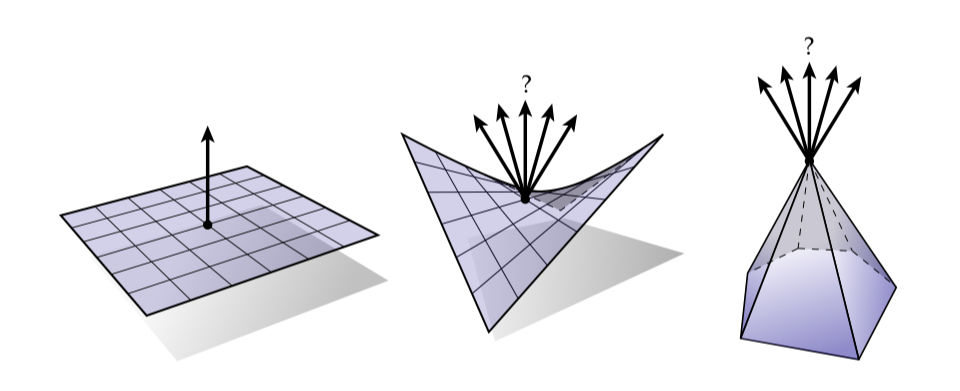## 向量面积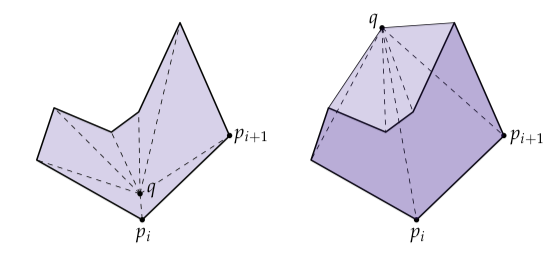$A = \intop_P dx \wedge dy$

$A = \frac{1}{2}\intop_P d(x \wedge dy) - d(y \wedge dx) = \frac{1}{2}\intop_{\partial P} x \wedge dy - y \wedge dx$

$\intop_{\partial P}x \wedge dy - y \wedge dx = \sum \intop_{e_{ij}} x\wedge dy - y \wedge dx$

\begin{aligned} \sum \intop_{e_{ij}} x \wedge dy - y \wedge dx &= \sum \frac{x_i + x_j}{2}(y_j - y_i) - \frac{y_i + y_j}{2}(x_j - x_i) \\ &= \frac{1}{2} \sum (p_i + p_j) \times (p_j - p_i) \\ &= \frac{1}{2} \sum p_i \times p_j - p_i \times p_i - p_j \times p_j - p_j \times p_i \\ &= \sum p_i \times p_j \end{aligned}

$A = \frac{1}{2} \sum_i p_i \times p_j$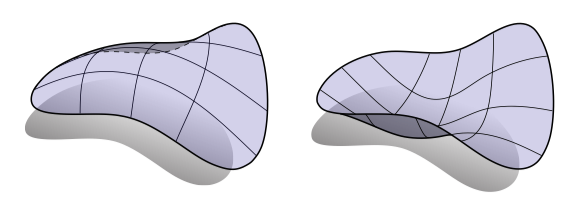$N_\mathcal{V} = \intop_M NdA$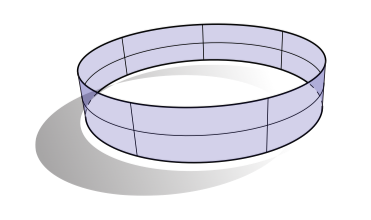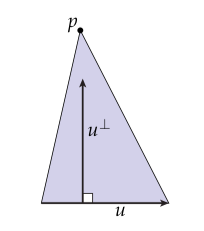## 面积梯度

$\nabla_p A = \sum_{\sigma} \nabla_p A_{\sigma}$

### 平均曲率向量

$\Delta f = 2 HN$

$\Delta \phi = \frac{d(d\phi(X))(X) + d(d\phi(\Upsilon))(\Upsilon)}{e^{2u}}$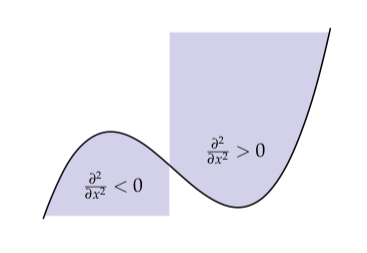## 体积梯度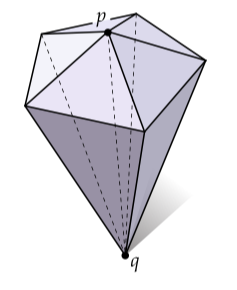$\mathcal{V} = \frac{1}{3}Ah$

$\nabla_p \mathcal{V} = \frac{1}{3}AN$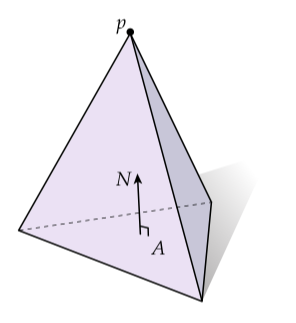$\nabla_p \mathcal{V} = \sum_i \mathcal{V}_i = \frac{1}{3} \sum_i A_i N_i$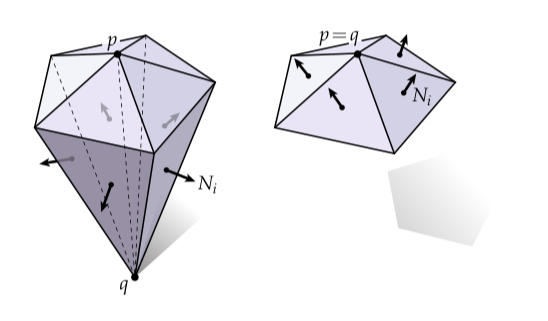## 其它的定义

### 统一加权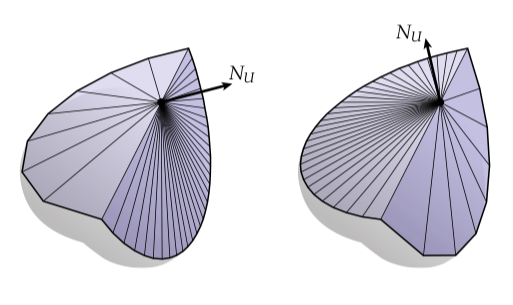$N_u \coloneqq \sum_i N_i$

### 顶角权重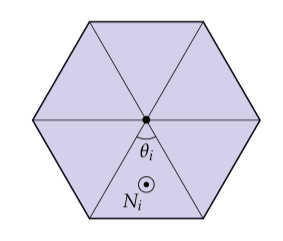$N_{\theta} \coloneqq \sum_i \theta_i N_i$

### 球雕刻的多面体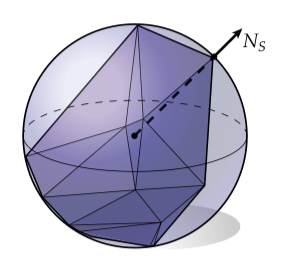$N_S = \frac{1}{c} \sum_{j=0}^{n-1} \frac{e_j \times e_{j+1}}{|e_j|^2 |e_{j+1}|^2}$